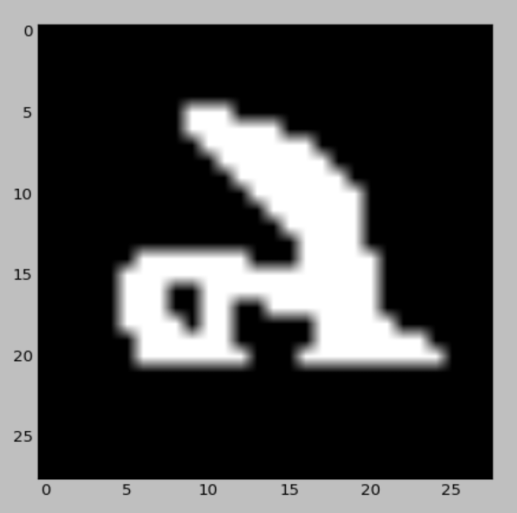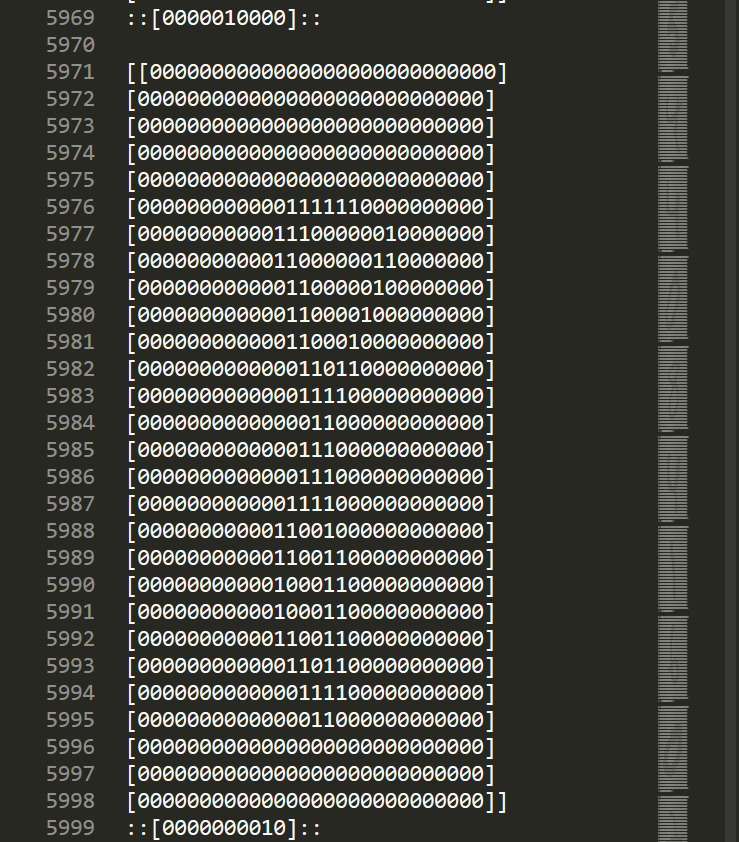## Classification Generator Training Attempt - Unconventional Neural Networks in Python and Tensorflow p.4

Hello and welcome to part 4 of our series of having some fun with neural networks, currently with generative networks. Wher we left off, we're building the dataset that we intend to use with our mnist generative model. We left off with quite a few questions. Typically, with ML algorithms, our data needs to be structured in a very specific way. With this generative network, and our goals, we actually have a lot of options for how we want to do this. The format can be anything, and our only goal is really to try to make this as easy as possible for the model to understand.

Right now, our images, being 28x28, are sequence lengths of 28x28, which means a full image would be 784 characters at a mimimum, unless we resize them. That said, our array has extra things like brackets, and spaces between each value. I think a good next step would be to remove the spaces. I cannot imagine any reason why these spaces are meaningful or why they'd be helpful to our model, I think they'll just waste our processing. Let's get rid of them! We should be able to just do:

`print(str(pixels).replace(' ',''))`

Making our full script:

```from tensorflow.examples.tutorials.mnist import input_data
import matplotlib.pyplot as plt
import numpy as np

# mnist.train, mnist.test, mnist.validation

batch_xs, batch_ys = mnist.train.next_batch(100)

data = np.rint(batch_xs).astype(int)
label = np.rint(batch_ys).astype(int)

pixels = data.reshape((28,28))

print(data)
print(label)
print(str(pixels).replace(' ',''))

plt.imshow(pixels, cmap='gray')
plt.show()```

Interestingly, the image I got this time was:The cleaned array is:

```[


























]```

At first glance, I have no idea what this is supposed to even be. A coiled snake?

The array is: `[0 0 1 0 0 0 0 0 0 0]` ... so it's a 2. Knowing this, I could see that, it's probably a badly scanned 2, where the bottom is merely chopped off.

Anyway, looks like our cleaning method worked, Next, let's clean the one-hot array:

`print(str(label).replace(' ',''))`

Now, for a 7, this is ``

Okay, fairly clean. Let's create a training file now. I think the one-hot array distinction is enough for the generative model to separate numbers. The order of the label and image data will depend on the task we're attempting. If we're attempting to do classification, then we'd want the image data first, then the label in the training data. If we wanted a generative model to take a number, 0-9, and draw that number for us, then we'd want the label array first.

I plan to do both, and I am equally curious about both, but let's try classification first, so let's put the image first, array last.

Let's also assume we were going to make this into a production-like model, we'd need some logic to know when the prediction was done, so I am thinking about using maybe a double colon to differentiate it. It may be the case that the length of the array is enough, I really have no idea. This is all guessing. So the model will be fed the image data, then we'll have it generate 16 or more characters to see the prediction.

So each training sample can be written out like:

`classify_data = "{}\n::{}::\n\n".format(str_img, str_label)`

Where it's first the image data, then a new line, then, encased in double colons, the label, then two new lines to separate sample sets.

We aren't going to do it yet, but to make the training set to generate a number based on input, we'd probably do:

`gen_to_data = "::{}::\n{}\n\n".format(str_label, str_img)`

Now, the full script up to this point is something like:

File name/location: `char-rnn-tensorflow-master/data/mnist/mnist-data-creation.py`

```from tensorflow.examples.tutorials.mnist import input_data
import matplotlib.pyplot as plt
import numpy as np

# mnist.train, mnist.test, mnist.validation
batch_xs, batch_ys = mnist.train.next_batch(10000)

with open('classify/input.txt','a') as f:
for i, data in enumerate(batch_xs):
data = np.rint(batch_xs[i]).astype(int)
label = np.rint(batch_ys[i]).astype(int)
pixels = data.reshape((28,28))
str_label = str(label).replace(' ','')
str_img = str(pixels).replace(' ','')
#gen_to_data = "::{}::\n{}\n\n".format(str_label, str_img)
classify_data = "{}\n::{}::\n\n".format(str_img, str_label)
#plt.imshow(pixels, cmap='gray')
#plt.show()
f.write(classify_data)```

Note: I changed the batch size to be 10,000, so we'll have 10K samples of data. I have no idea if this is a worthy size of data or not.

Run this, and you should get the input.txt file. Open it to make sure it looks something like:If you're not using sublime text, don't mind the right-hand side there, that just shows a zoomed-out view of the entire file.

Alright, this all looks good to me, let's train! If possible, we'd probably like the sequence to be quite large.

`python train.py --data_dir=data/mnist/classify --rnn_size=128 --num_layers=3 --batch_size=28 --seq_length=750`

I think sequence length is definitely the most important thing here, so I am first trying the above to see if it will fit in my GPU. I lowered back down to 128 nodes per layer, but still trying 3 layers. I think we could probably go with a smaller sequence, such as 250, since thatd still be a sliding window of about a 3rd of the image anyway, it should still be totally possible to classify like this, but we'd be far more dependent on the generative model to do more than just be able to classify, it'd need to also be able to generate numbers by guessing the number the whole time. I think it'd be more likely to go wrong, but, then again, I really don't know. Too large and it might draw numbers based on the previous numbers. Did I mention this is all a test?

The above does fit, but it's REALLY dreadfully slow. I am going to bump down to 250, and maybe try out a longer sequence some time when I have over-night to train. I am going to re-try with:

`python train.py --data_dir=data/mnist/classify --rnn_size=128 --num_layers=3 --batch_size=50 --seq_length=250`

That's quite a bit faster, I think I will go with that for now. Okay, I am going to eat something. See you in the next part!

Note: during the filming of this part, I decided to try a different method for the classification part, which saw a slight improvement over the above method. Here's the difference:

```from tensorflow.examples.tutorials.mnist import input_data
import matplotlib.pyplot as plt
import numpy as np

# mnist.train .test  .validate
batch_xs, batch_ys = mnist.train.next_batch(10)

with open("classify/input.txt","a") as f:
for i, data, in enumerate(batch_xs):
data = np.rint(batch_xs[i]).astype(int)
label = np.rint(batch_ys[i]).astype(int)
pixels = data.reshape((28,28))
index_value = np.argmax(label)
new_label = np.array(100*[index_value]).reshape((10,10))

str_img = str(pixels).replace(" ","")
str_label = str(new_label).replace(" ","")

classify_data = "{}\n:{}:\n\n".format(str_img, str_label)
f.write(classify_data)```

Basically, the label, rather than being one row and one-hot, is instead just a serious of the number we want in repepetion. My line of thinking there is that the model will have more than just one shot to get things right, and will be easier to learn in training.

The next tutorial:• Generative Model Basics (Character-Level) - Unconventional Neural Networks in Python and Tensorflow p.1

• Generating Pythonic code with Character Generative Model - Unconventional Neural Networks in Python and Tensorflow p.2

• Generating with MNIST - Unconventional Neural Networks in Python and Tensorflow p.3

• Classification Generator Training Attempt - Unconventional Neural Networks in Python and Tensorflow p.4
• Classification Generator Testing Attempt - Unconventional Neural Networks in Python and Tensorflow p.5

• Drawing a Number by Request with Generative Model - Unconventional Neural Networks in Python and Tensorflow p.6

• Deep Dream - Unconventional Neural Networks in Python and Tensorflow p.7

• Deep Dream Frames - Unconventional Neural Networks in Python and Tensorflow p.8

• Deep Dream Video - Unconventional Neural Networks in Python and Tensorflow p.9

• Doing Math with Neural Networks - Unconventional Neural Networks in Python and Tensorflow p.10

• Doing Math with Neural Networks testing addition results - Unconventional Neural Networks in Python and Tensorflow p.11

• Complex Math - Unconventional Neural Networks in Python and Tensorflow p.12